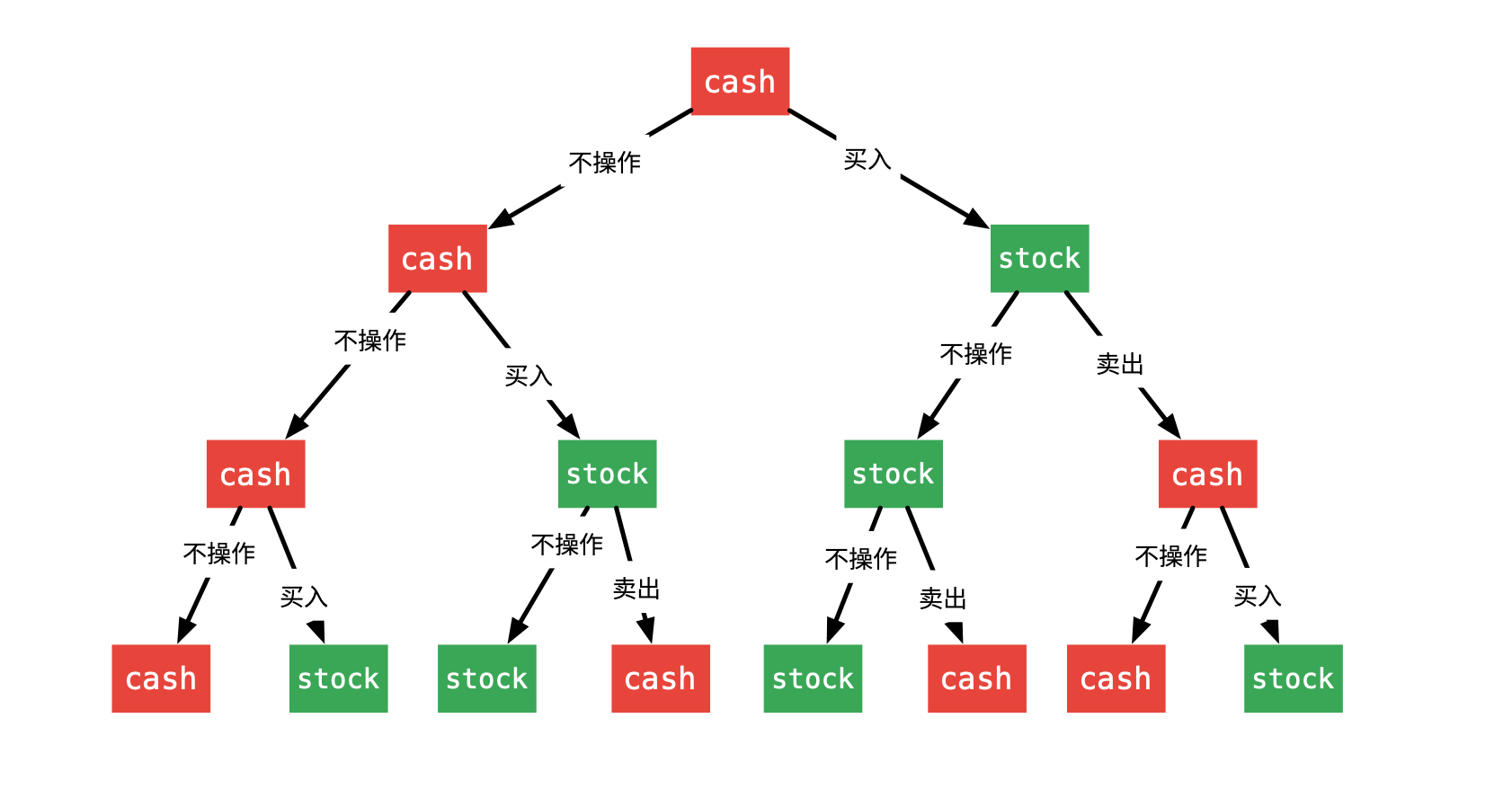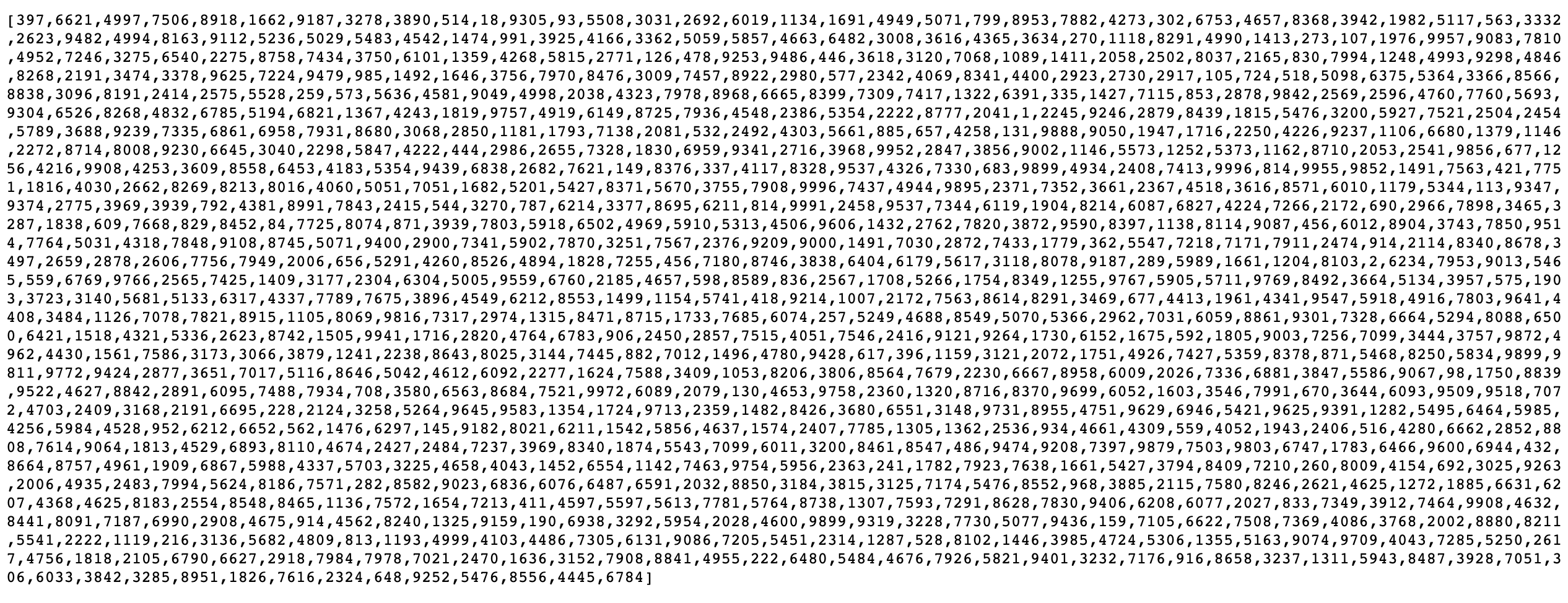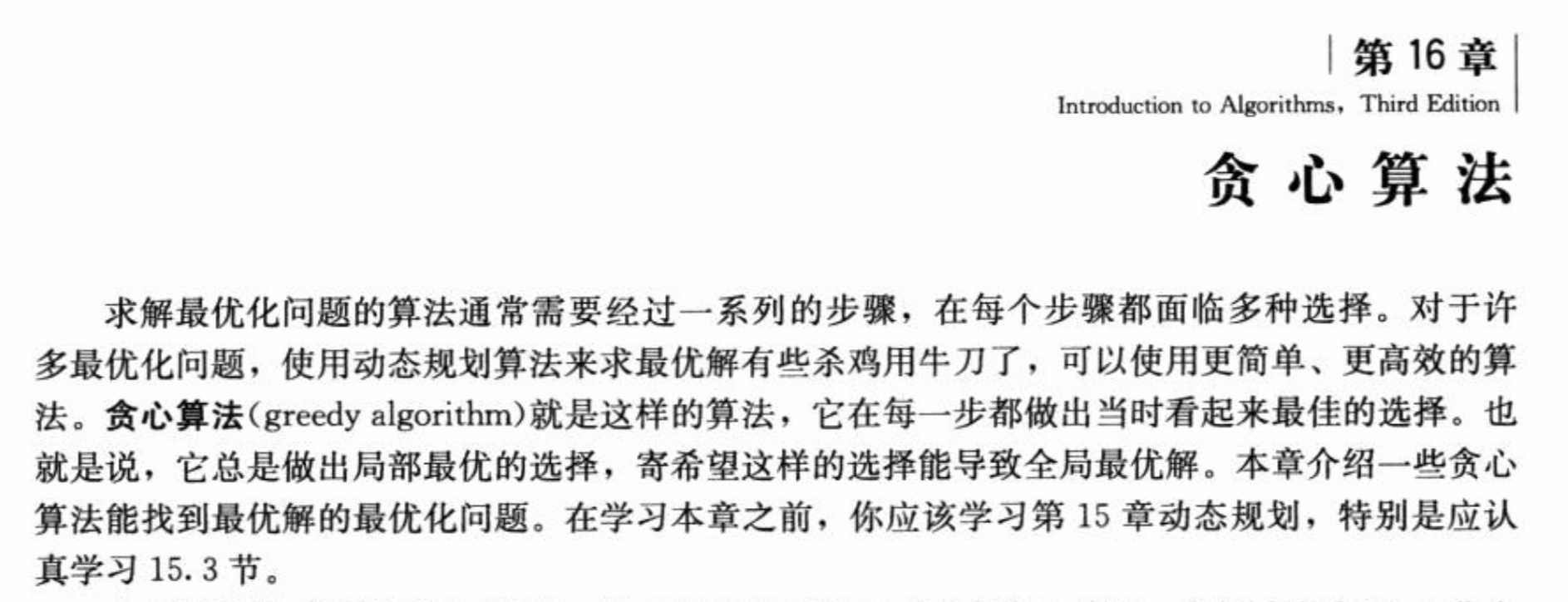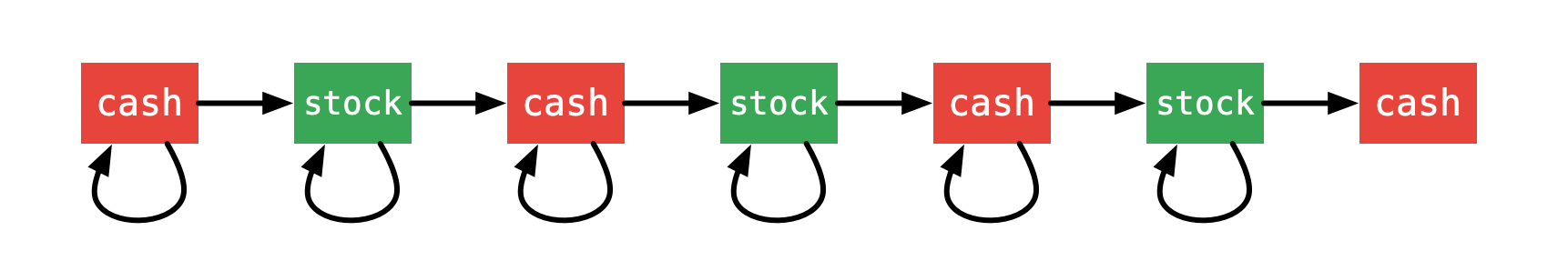# 「力扣」第 122 题：买卖股票的最佳时机 II（简单）

## 「力扣」第 122 题：买卖股票的最佳时机 II（简单）

输入: [7,1,5,3,6,4]

随后，在第 4 天（股票价格 = 3）的时候买入，在第 5 天（股票价格 = 6）的时候卖出, 这笔交易所能获得利润 = 6-3 = 3 。

输入: [1,2,3,4,5]

注意你不能在第 1 天和第 2 天接连购买股票，之后再将它们卖出。
因为这样属于同时参与了多笔交易，你必须在再次购买前出售掉之前的股票。

输入: [7,6,4,3,1]

### 方法一：暴力搜索{:width=500}

public class Solution {

private int res;

public int maxProfit(int[] prices) {
int len = prices.length;
if (len < 2) {
return 0;
}
this.res = 0;
dfs(prices, 0, len, 0, res);
return this.res;
}

/**
* @param prices 股价数组
* @param index  当前是第几天，从 0 开始
* @param status 0 表示不持有股票，1表示持有股票，
* @param profit 当前收益
*/
private void dfs(int[] prices, int index, int len, int status, int profit) {

if (index == len) {
this.res = Math.max(this.res, profit);
return;
}

dfs(prices, index + 1, len, status, profit);

if (status == 0) {
// 可以尝试转向 1
dfs(prices, index + 1, len, 1, profit - prices[index]);

} else {
// 此时 status == 1，可以尝试转向 0
dfs(prices, index + 1, len, 0, profit + prices[index]);
}
}
}### 方法二：贪心算法

• 从第 i 天（这里 i >= 1）开始，与第 i - 1 的股价进行比较，如果股价有上升（严格上升），就将升高的股价（ prices[i] - prices[i- 1] ）记入总利润，按照这种算法，得到的结果就是符合题意的最大利润。

1、该算法仅可以用于计算，但计算的过程并不是真正交易的过程，但可以用贪心算法计算题目要求的最大利润。下面说明这个等价性：以 [1, 2, 3, 4] 为例，这 4 天的股价依次上升，按照贪心算法，得到的最大利润是：

res =  (prices - prices) + (prices - prices) + (prices - prices)
=  prices - prices

2、解释一下它为什么叫 “贪心算法”{:width=560}

“贪心算法” 在每一步总是做出在当前看来最好的选择。

• “贪心算法” 和 “动态规划”、“回溯搜索” 算法一样，完成一件事情，是分步决策的；
• “贪心算法” 在每一步总是做出在当前看来最好的选择，我是这样理解 “最好” 这两个字的意思：
1. “最好” 的意思往往根据题目而来，可能是 “最小”，也可能是 “最大”；

2. 贪心算法和动态规划相比，它既不看前面（也就是说它不需要从前面的状态转移过来），也不看后面（无后效性，后面的选择不会对前面的选择有影响），因此贪心算法时间复杂度一般是线性的，空间复杂度是常数级别的。

3. 这道题 “贪心” 的地方在于，对于 “今天的股价 - 昨天的股价”，得到的结果有 3 种可能：（1）正数（2）0（3）负数。

public class Solution {

public int maxProfit(int[] prices) {
int res = 0;
int len = prices.length;
for (int i = 0; i < len - 1; i++) {
int diff = prices[i + 1] - prices[i];
if (diff > 0) {
res += diff;
}
}
return res;
}
}

• 时间复杂度：$O(N)$，这里 $N$ 表示股价数组的长度；
• 空间复杂度：$O(1)$。

Java 代码：

public class Solution {

public int maxProfit(int[] prices) {
int res = 0;
int len = prices.length;
for (int i = 0; i < len - 1; i++) {
res += Math.max(prices[i + 1] - prices[i], 0);
}
return res;
}
}

1、如果可行解在 “贪心算法” 的基础上，选择了差分为 0 的项，得到的结果与“贪心算法”得到的结果一样，因此加上差分为 0 的项不会比“贪心算法”得到的结果更好；

2、如果可行解在 “贪心算法” 的基础上，选择了差分为负数的项，加上一个负数得到的结果一定比 “贪心算法” 得到的结果要少，加上差分为负数的项，一定比 “贪心算法” 得到的结果更少；

3、如果可行解在 “贪心算法” 的基础上，去掉了任何一个差分为正数的项，同上，得到的结果一定比 “贪心算法” 得到的结果要小，因此，“贪心算法” 的所有组成项不能删去任何一个。

### 方法三：动态规划

#### 第 1 步：定义状态

• 第一维 i 表示索引为 i 的那一天（具有前缀性质，即考虑了之前天数的收益）能获得的最大利润；
• 第二维 j 表示索引为 i 的那一天是持有股票，还是持有现金。这里 0 表示持有现金（cash），1 表示持有股票（stock）。

#### 第 2 步：思考状态转移方程

• 状态从持有现金（cash）开始，到最后一天我们关心的状态依然是持有现金（cash）；
• 每一天状态可以转移，也可以不动。状态转移用下图表示：{:width=500}

（状态转移方程写在代码中）

• 因为不限制交易次数，除了最后一天，每一天的状态可能不变化，也可能转移；
• 写代码的时候，可以不用对最后一天单独处理，输出最后一天，状态为 0 的时候的值即可。

#### 第 3 步：确定起始

• 如果什么都不做，dp = 0
• 如果买入股票，当前收益是负数，即 dp = -prices[i]

#### 第 4 步：确定终止

public class Solution {

public int maxProfit(int[] prices) {
int len = prices.length;
if (len < 2) {
return 0;
}

// 0：持有现金
// 1：持有股票
// 状态转移：0 → 1 → 0 → 1 → 0 → 1 → 0
int[][] dp = new int[len];

dp = 0;
dp = -prices;

for (int i = 1; i < len; i++) {
// 这两行调换顺序也是可以的
dp[i] = Math.max(dp[i - 1], dp[i - 1] + prices[i]);
dp[i] = Math.max(dp[i - 1], dp[i - 1] - prices[i]);
}
return dp[len - 1];
}
}

• 时间复杂度：$O(N)$，这里 $N$ 表示股价数组的长度。
• 空间复杂度：$O(N)$，虽然是二维数组，但是第二维是常数，与问题规模无关。

public class Solution {

public int maxProfit(int[] prices) {
int len = prices.length;
if (len < 2) {
return 0;
}

// cash：持有现金
// hold：持有股票
// 状态数组
// 状态转移：cash → hold → cash → hold → cash → hold → cash
int[] cash = new int[len];
int[] hold = new int[len];

cash = 0;
hold = -prices;

for (int i = 1; i < len; i++) {
// 这两行调换顺序也是可以的
cash[i] = Math.max(cash[i - 1], hold[i - 1] + prices[i]);
hold[i] = Math.max(hold[i - 1], cash[i - 1] - prices[i]);
}
return cash[len - 1];
}
}

#### 第 5 步：考虑状态压缩

public class Solution {

public int maxProfit(int[] prices) {
int len = prices.length;
if (len < 2) {
return 0;
}

// cash：持有现金
// hold：持有股票
// 状态转移：cash → hold → cash → hold → cash → hold → cash

int cash = 0;
int hold = -prices;

int preCash = cash;
int preHold = hold;
for (int i = 1; i < len; i++) {
cash = Math.max(preCash, preHold + prices[i]);
hold = Math.max(preHold, preCash - prices[i]);

preCash = cash;
preHold = hold;
}
return cash;
}
}

• 时间复杂度：$O(N)$，这里 $N$ 表示股价数组的长度。
• 空间复杂度：$O(1)$，分别使用两个滚动变量，将一维数组状态压缩到常数。

上一篇「力扣」第 123 题：买卖股票的最佳时机 III（困难）
「力扣」第 123 题：买卖股票的最佳时机 III（困难） 链接 题解链接 给定一个数组，它的第 i 个元素是一支给定的股票在第 i 天的价格。 设计一个算法来计算你所能获取的最大利润。你最多可以完成 两笔 交易。 注意: 你不能同时参
2018-04-20「力扣」第 121 题：买卖股票的最佳时机（简单）
「力扣」第 121 题：买卖股票的最佳时机（简单） 链接 题解链接 给定一个数组，它的第 i 个元素是一支给定股票第 i 天的价格。 如果你最多只允许完成一笔交易（即买入和卖出一支股票），设计一个算法来计算你所能获取的最大利润。 注意你
2018-04-18
目录